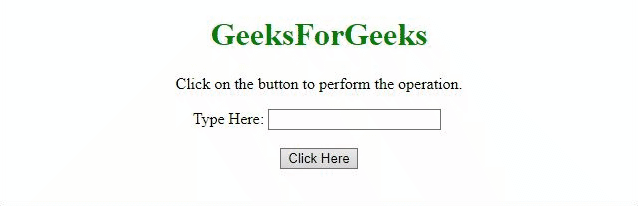# How to convert first letter of a string to upper case using jQuery ?

The task is to capitalize the first letter of string without using toUpperCase() method with the help of jQuery. There are two approaches that are discussed below:

Approach 1: In this example, the css() method is used to used to set the text-transform property value to capitalize.

Example:

 `  ` `<``html``>  ` ` `  `<``head``>  ` `    ``<``title``>  ` `        ``How to convert first letter of a ` `        ``string to upper case using jQuery? ` `    `` ` `     `  `    ``<``script` `src``= ` `"https://ajax.googleapis.com/ajax/libs/jquery/3.4.0/jquery.min.js"``> ` `    `` ` `  ` ` `  `<``body` `style` `= ``"text-align:center;"``>  ` `     `  `    ``<``h1` `style` `= ``"color:green;"` `>  ` `        ``GeeksForGeeks  ` `    `` ` `     `  `    ``<``p``> ` `        ``Click on the button to ` `        ``perform the operation. ` `    `` ` `     `  `    ``Type Here: <``input` `id` `= ``"input"``/> ` `    ``<``br``><``br``> ` `     `  `    ``<``button` `onclick` `= ``"GFG_Fun()"``> ` `        ``Click Here ` `    `` ` `     `  `    ``<``p` `id` `= ``"GFG"``> ` `     `  `    ``<``script``> ` `        ``var geeks = document.getElementById('GFG'); ` `         `  `        ``function GFG_Fun() { ` `            ``\$('#input').css('textTransform', 'capitalize'); ` `            ``geeks.innerHTML = "Text is capitalized"; ` `        ``} ` `    ``  ` `  ` ` `  ` `

Output:Approach 2: In this example, we are using CSS property to perform the operation. A new ID has been added to the element which sets the property text-transform to capitalize.

Example:

 `  ` `<``html``>  ` ` `  `<``head``>  ` `    ``<``title``>  ` `        ``How to convert first letter of a ` `        ``string to upper case using jQuery? ` `    `` ` `     `  `    ``<``script` `src``= ` `"https://ajax.googleapis.com/ajax/libs/jquery/3.4.0/jquery.min.js"``> ` `    `` ` `     `  `    ``<``style``> ` `        ``#capital { ` `            ``text-transform: capitalize; ` `        ``} ` `    `` ` `  ` ` `  `<``body` `style` `= ``"text-align:center;"``>  ` `     `  `    ``<``h1` `style` `= ``"color:green;"` `>  ` `        ``GeeksForGeeks  ` `    `` ` `     `  `    ``<``p``> ` `        ``Click on the button to ` `        ``perform the operation. ` `    `` ` `     `  `    ``Type Here: <``input` `id` `= ``"input"``/> ` `    ``<``br``><``br``> ` `     `  `    ``<``button` `onclick` `= ``"GFG_Fun()"``> ` `        ``Click Here ` `    `` ` `     `  `    ``<``p` `id` `= ``"GFG"``> ` `     `  `    ``<``script``> ` `        ``var geeks = document.getElementById('GFG'); ` `         `  `        ``function GFG_Fun() { ` `            ``\$('#input').attr('id', 'capital'); ` `            ``geeks.innerHTML = "Text is capitalized"; ` `        ``} ` `    ``  ` `  ` ` `  ` `

Output:My Personal Notes arrow_drop_upCheck out this Author's contributed articles.

If you like GeeksforGeeks and would like to contribute, you can also write an article using contribute.geeksforgeeks.org or mail your article to contribute@geeksforgeeks.org. See your article appearing on the GeeksforGeeks main page and help other Geeks.

Please Improve this article if you find anything incorrect by clicking on the "Improve Article" button below.